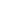We condemn the war and support Ukraine in its struggle for democratic values.
We also encourage you to join the #StandWithUkraine movement by making a donation at this linkForecasting for M&L Manufacturing Essay

### Q1. What are some of the potential benefits of a more formalized approach to forecasting?

The potential benefits for M&L manufacturing are:

• Proper production planning in future of two of its most valuable and profitable product lines;
• Fewer stock-outs in retail stores;
• Less uncertainty with regard to unexpected orders and production failures;
• Proper inventory management and control over the stock levels;
• Better knowledge regarding which products to continue selling and discontinue selling.

Calculate the price### Q2. Prepare a weekly forecast for the next four weeks of each product. Briefly explain why you chose the methods you used.

#### Product 1

The demand for product one has risen over the period of 14 weeks which depicts that demand can be expected to rise further in the coming four weeks. The pattern can be illustrated using a line graph, as shown in figure 1, below.

Based on the shape of the graph, it can be stated that, except for the unusual order of 90 units in the seventh week, the demand has risen over the period in a rising trend. Because of the size of the order the graph gets put off from an otherwise linear pattern of the rising demand.

In order to forecast demand of Product, the following features of the demand of the product need to be noted:

• The demand has risen steadily over the period of 14 weeks;
• The slight deviation in between has been caused by an unusual order which is not expected in future sales.

The forecasting method most suited to the nature of the demand of Product 1 is ‘Trend Analysis’ using a regression model. Trend analysis is a method of forecasting whereby the data is assumed to show a linear behaviour over a long period of time and based on the linear nature of the growth in sales, a linear equation, obtained through regression analysis is established to make predictions about sales and demand in future and make forecast. The method is suited for long term forecasts as well as short term forecasts.

Using the data obtained from the case study which has been presented in a graph form in Figure 1, regression analysis is conducted to formulate a linear equation based on the existing demand trend. This is done using calculations.

However, rather than manual calculations, MS EXCEL provides a faster mode of calculating and plotting the historic demand figures to develop a regression trend line.. EXCEL allows creating a chart of the demand units against weeks. The chart generates a linear representation of the data which can be further projected for forecasting future sales of the next four weeks using a trend line. EXCEL allows the linear equation to be developed for the trend line as well as produces the figure of R-squared, denoted by r in the above set of formulas.

In the Trend Analysis method of forecasting sales, R-squared value allows estimating the accuracy of the forecast. It represents the deviation that the trend line has from the data. The larger this figure is and the closer it is to 1, the more accurate the forecasts are as they follow the trend in the sales data.

The linear Trend Analysis in the form of a graph is shown in Figure 2, below.

The trend line equation generated by EXCEL is y = 3.457x + 48.28, were y represents the units of the product and x represents the week. This equation shows the total demand never falls below 48 units and the company can expect this much minimum demand to exist in the coming weeks even in the hard times. The r-squared is 0.889 which is highly close to 1 depicting that the trend line follows the pattern of the demand data. This figure is mainly due to the unusual order placed by a customer of 90 units. Therefore, it can be concluded that the Trend Analysis is suitable for the forecasting of the future demand of Product 1 produced by the company as it shows strong accuracy.

## Our features300 words per page instead of 280Discount SystemVip ServicesRevision within 2 days (on demand)Affiliate ProgramPlagiarism-free papers

#### Product 2

The data present in the case study is used to create a graph using EXCEL, which is shown in Figure 3, below.

Based on the nature of the spread of the data, it can be safely said that the data shows variations and seldom progresses in a linear fashion.

The exponential smoothening method of forecasting is most suitable to determine the future demand of the product because it would allow ‘smoothening’ these variations. It is a simple method compared with the regression model and places value in the most recent values of the demand of the product to predict the future demand values.

EXCEL is used to generate the exponential smoothening graph and equation necessary to forecast the future demand of Product 2. The findings are shown in Figure 4, below.

The exponential equation drawn by EXCEL for the data is y + 40.04e0.008x.

The forecasted values of Product 1 using linear Trend Analysis and Product 2 using Exponential Smoothening method are shown below in Table 1.

 Table 1: Forecasted Demand of Product 1 and Product 2 Week Product 1 Product 2 15 100 45 16 103 46 17 107 46.5 18 110 47